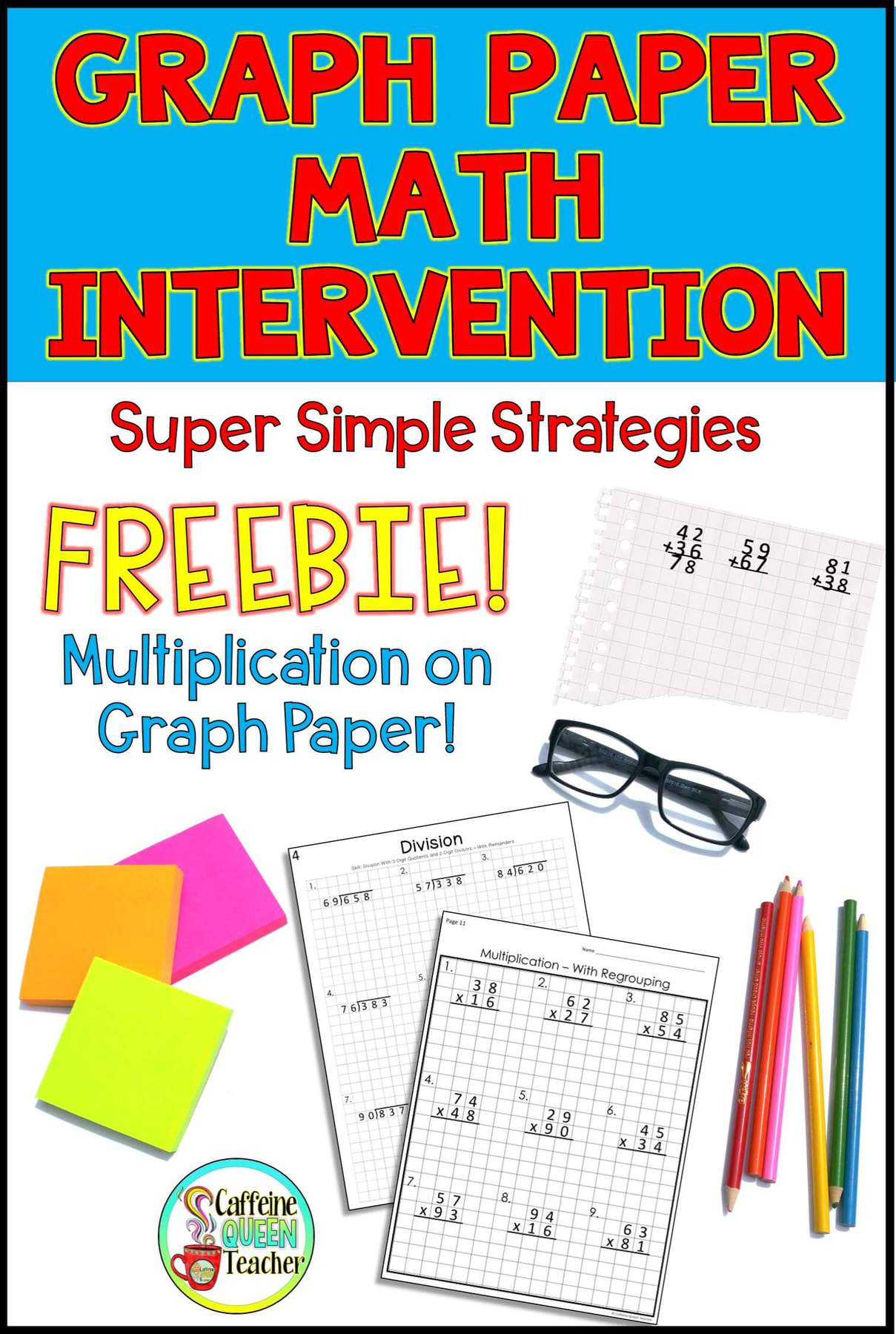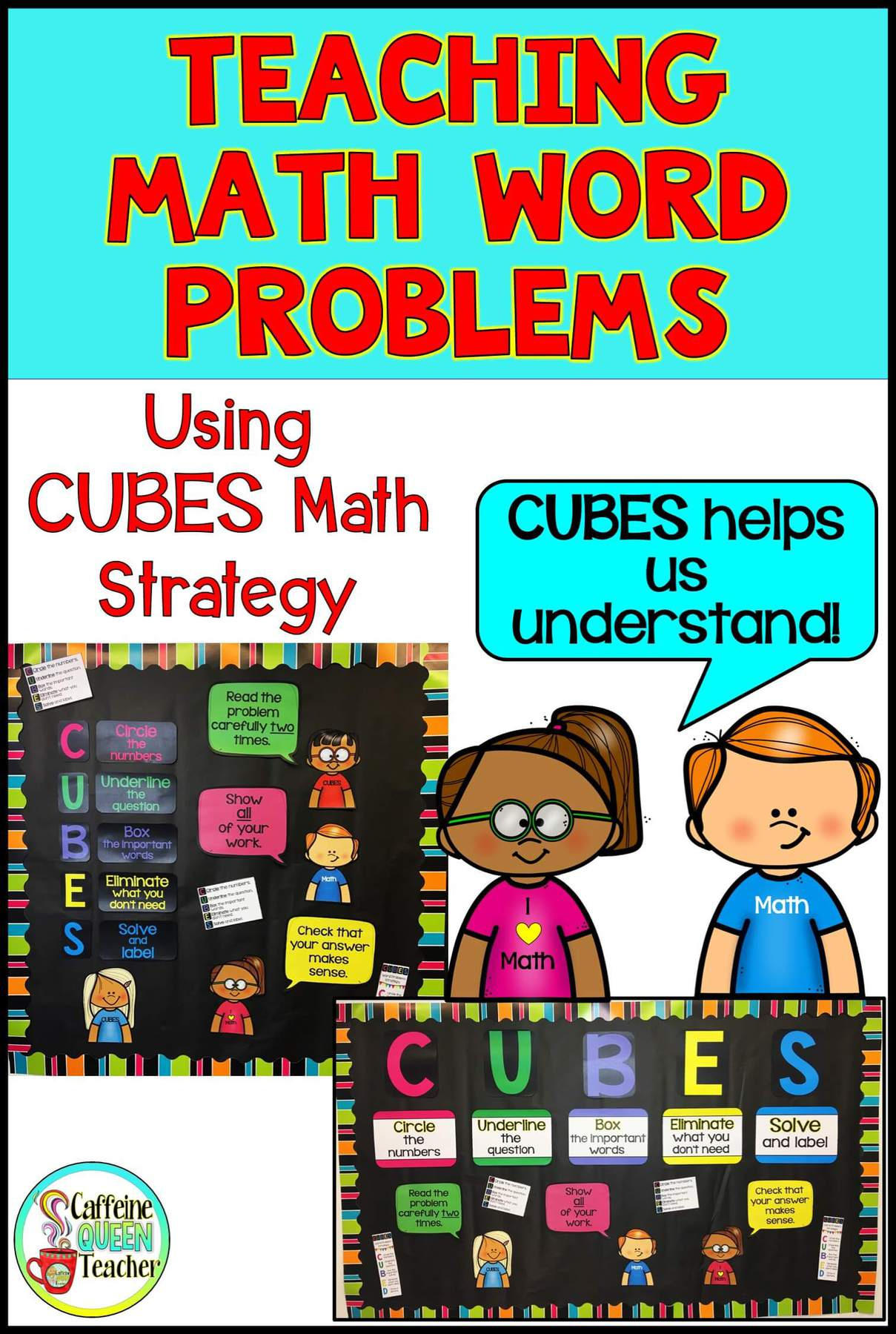# Category: Sticky### Graph Paper Math Intervention

Don’t overlook graph paper if your students are struggling with math! It’s an effective yet often overlooked way to scaffold, differentiate, and help students master arithmetic. A simple sheet of graph paper can improve students’ math arithmetic skills for addition, subtraction, multiplication, and division. – And fractions, decimals, equations, and